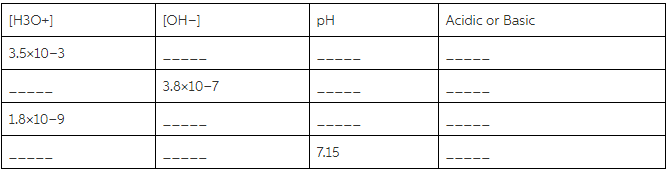# Problem: Complete the following table. All solutions are at 25°C. (Up to two significant figures)

###### FREE Expert Solution

To complete the table:

$\overline{){\mathbf{pH}}{\mathbf{+}}{\mathbf{pOH}}{\mathbf{=}}{\mathbf{14}}}$

$\overline{)\left[{\mathbf{H}}_{\mathbf{3}}{\mathbf{O}}^{\mathbf{+}}\right]{\mathbf{=}}{\mathbf{10}}^{\mathbf{-}\mathbf{pH}}}$ $\overline{)\left[{\mathbf{OH}}^{\mathbf{-}}\right]{\mathbf{=}}{{\mathbf{10}}}^{\mathbf{-}\mathbf{pOH}}}$

$\overline{){{\mathbf{K}}}_{{\mathbf{w}}}{\mathbf{=}}\left[{\mathbf{H}}_{\mathbf{3}}{\mathbf{O}}^{\mathbf{+}}\right]\left[{\mathbf{OH}}^{\mathbf{-}}\right]}$

Kw = 1.0x10-14 at T = 25°C

Recall that:

• pH < 7  acidic
• pH > 7  → basic
83% (44 ratings)###### Problem Details

Complete the following table. All solutions are at 25°C. (Up to two significant figures)